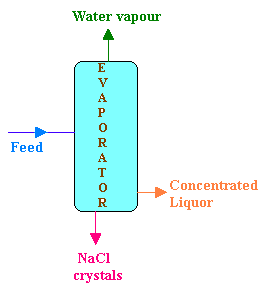### Evaporation

An evaporator is fed continuously with 25 kg/hr of a solution which contains 10% NaCl, 10% NaOH and 80% H2O. During evaporation, H2O is removed from the solution and NaCl precipitates as crystals which is settled and removed. The concentrated liquor leaving the evaporator contains 50% NaOH, 2% NaCl and 48% H2O. Calculate

(i)Weight of salt precipitated per hour. (6)
(ii) Weight of concentrated liquor leaving per hour. (6)

Calculations:Data:

Flow rate of feed = 25 kg/hr

Composition of feed (F):

NaCl 10%

NaOH 10%

H2O 80%

Composition of concentrated liquor (P):

NaCl 2%

NaOH 48%

H2O 50%

Balance for NaOH:

NaOH entering the in feed = NaOH leaving in concentrated liquor

i.e., 0.1 x 25 = 0.50 P

Therefore, the mass flow rate of concentrated liquor (P) = 0.1 x 25 / 0.50 = 5 kg/hr

Balance for NaCl:

NaCl entering in the feed = 25 x 0.1 = 2.5 kg/hr

The entering NaCl is coming out as crystals and in the concentrated liquor.

NaCl leaving in the concentrated liquor = 0.02 P = 0.02 x 5 = 0.1 kg/hr

Therefore,

NaCl precipitated = 2.5 - 0.1 = 2.4 kg/hr